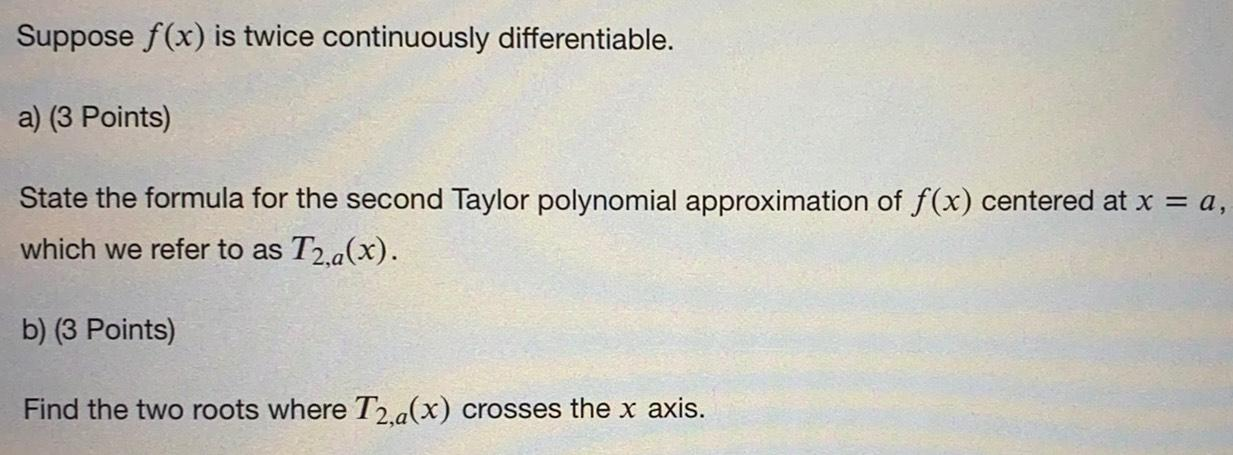Question:

# Suppose f(x) is twice continuously differentiable. a) (3 Points) State the formula for the second Taylor polynomial approximatioSuppose f(x) is twice continuously differentiable. a) (3 Points) State the formula for the second Taylor polynomial approximation of f(x) centered at x = a, which we refer to as T2,a(x). b) (3 Points) Find the two roots where T2,a(x) crosses the x axis.